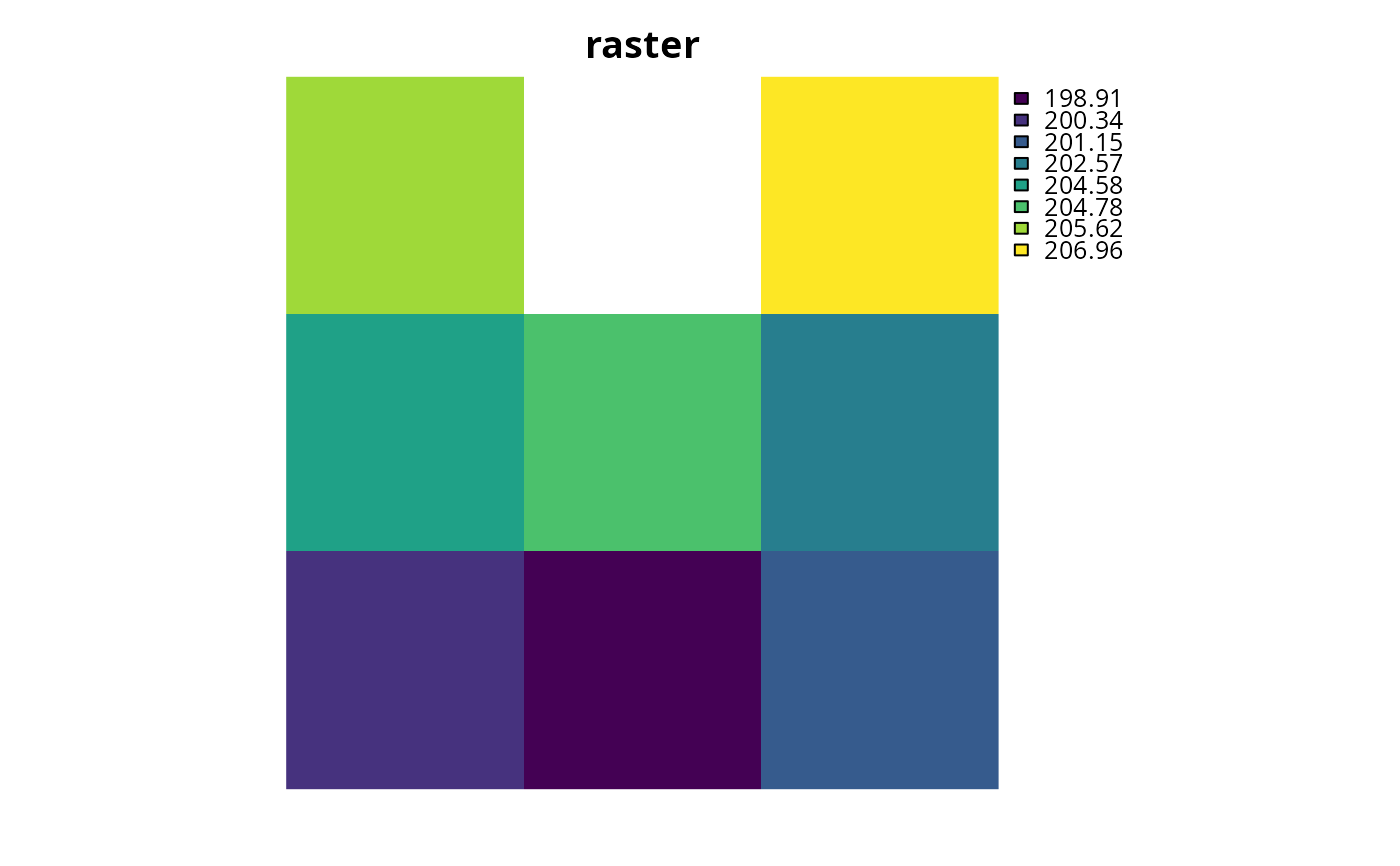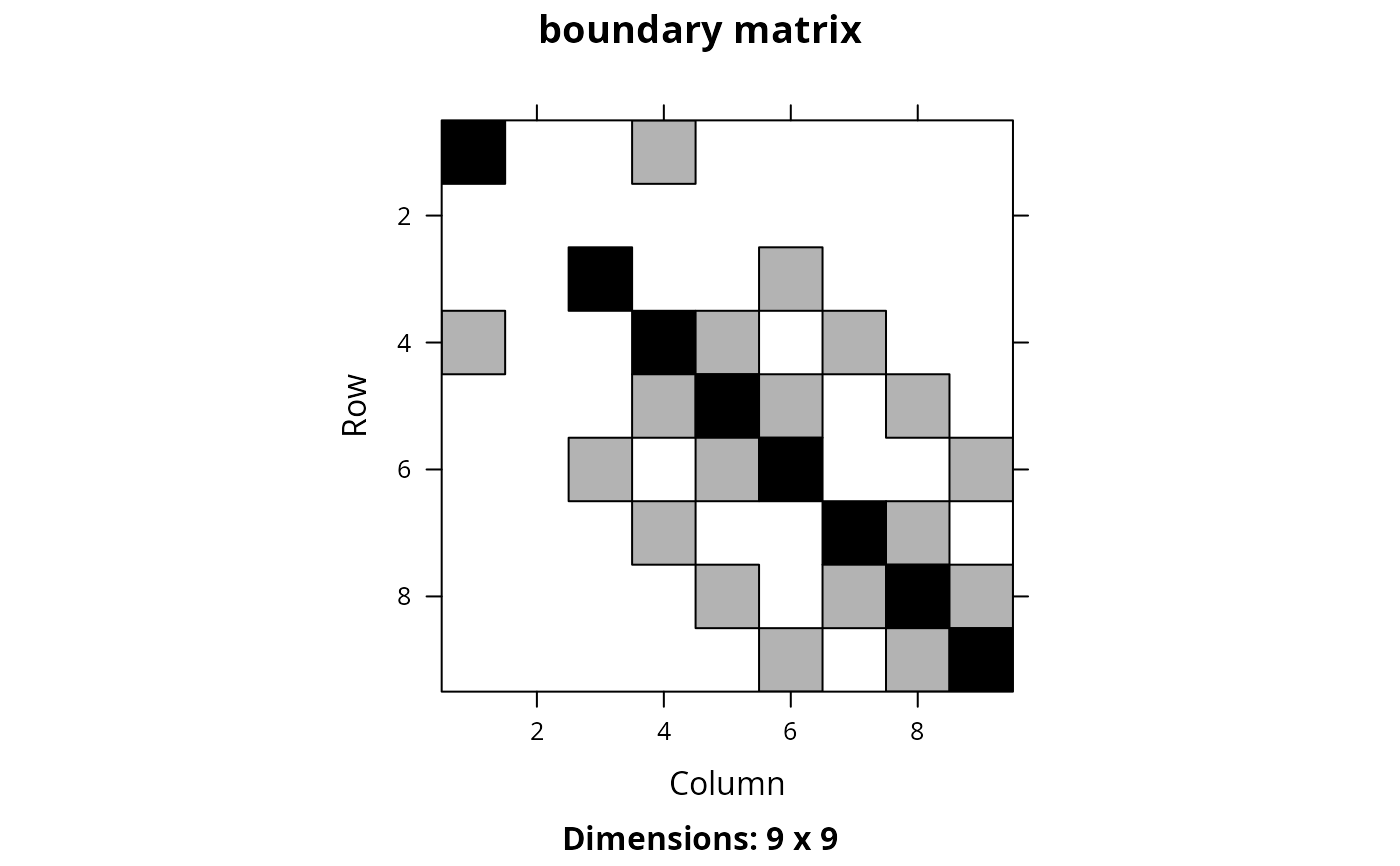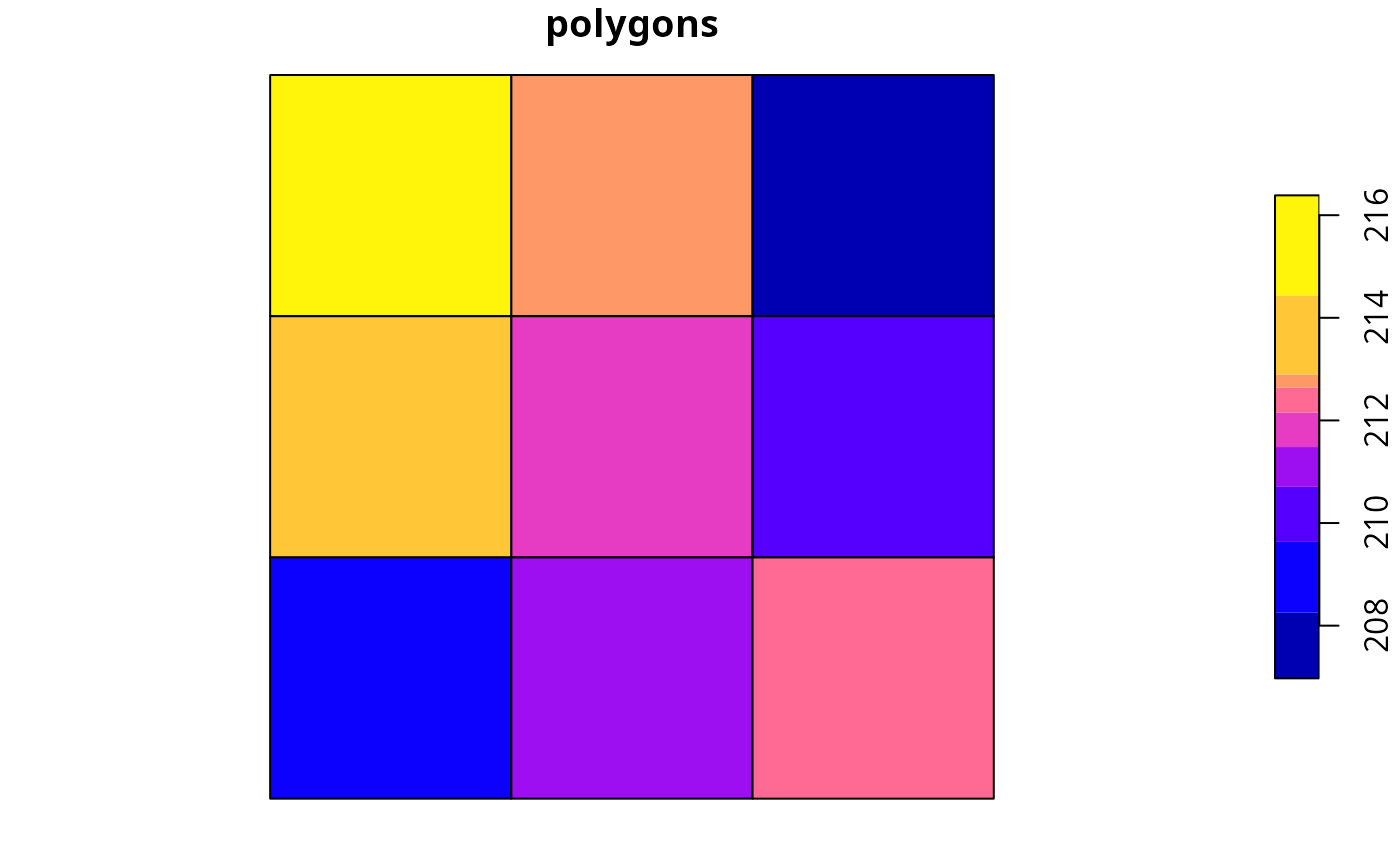Generate a matrix describing the amount of shared boundary length between different planning units, and the amount of exposed edge length each planning unit exhibits.

boundary_matrix(x, str_tree)

# S3 method for Raster
boundary_matrix(x, str_tree = FALSE)

# S3 method for SpatialPolygons
boundary_matrix(x, str_tree = FALSE)

# S3 method for SpatialLines
boundary_matrix(x, str_tree = FALSE)

# S3 method for SpatialPoints
boundary_matrix(x, str_tree = FALSE)

# S3 method for sf
boundary_matrix(x, str_tree = FALSE)

# S3 method for default
boundary_matrix(x, str_tree = FALSE)

## Arguments

x

Raster, SpatialLines, SpatialPolygons, sf::sf() object representing planning units. If x is a Raster object then it must have only one layer.

str_tree

logical should a GEOS STRtree structure be used to to pre-process data? If TRUE, then the experimental rgeos::gUnarySTRtreeQuery() function will be used to pre-compute which planning units are adjacent to each other and potentially reduce the processing time required to generate the boundary matrices. This argument is only used when the planning unit data are vector-based polygons (i.e., sp::SpatialPolygonsDataFrame() objects). Note that using TRUE may crash Mac OSX systems. The default argument is FALSE.

## Value

dsCMatrix symmetric sparse matrix object. Each row and column represents a planning unit. Cells values indicate the shared boundary length between different pairs of planning units.

## Details

This function returns a dsCMatrix symmetric sparse matrix. Cells on the off-diagonal indicate the length of the shared boundary between two different planning units. Cells on the diagonal indicate length of a given planning unit's edges that have no neighbors (e.g., for edges of planning units found along the coastline). This function assumes the data are in a coordinate system where Euclidean distances accurately describe the proximity between two points on the earth. Thus spatial data in a longitude/latitude coordinate system (i.e., WGS84) should be reprojected to another coordinate system before using this function. Note that for Raster objects boundaries are missing for cells that have NA values in all cells.

## Examples

# load data
data(sim_pu_raster, sim_pu_polygons)

# subset data to reduce processing time
r <- crop(sim_pu_raster, c(0, 0.3, 0, 0.3))
ply <- sim_pu_polygons[c(1:2, 10:12, 20:22), ]
ply2 <- st_as_sf(ply)

# create boundary matrix using raster data
bm_raster <- boundary_matrix(r)

# create boundary matrix using polygon (Spatial) data
bm_ply1 <- boundary_matrix(ply)

# create boundary matrix using polygon (sf) data
bm_ply2 <- boundary_matrix(ply2)

# create boundary matrix with polygon (Spatial) data and GEOS STR query trees
# to speed up processing
bm_ply3 <- boundary_matrix(ply, TRUE)

# plot raster and boundary matrix
# \dontrun{
par(mfrow = c(1, 2))
plot(r, main = "raster", axes = FALSE, box = FALSE)
plot(raster(as.matrix(bm_raster)), main = "boundary matrix",
axes = FALSE, box = FALSE)# }
# plot polygons and boundary matrices
# \dontrun{
par(mfrow = c(1, 3))
plot(r, main = "polygons (Spatial)", axes = FALSE, box = FALSE)
plot(raster(as.matrix(bm_ply1)), main = "boundary matrix", axes = FALSE,
box = FALSE)
plot(r, main = "polygons (sf)", axes = FALSE, box = FALSE)plot(raster(as.matrix(bm_ply2)), main = "boundary matrix", axes = FALSE,
box = FALSE)
plot(raster(as.matrix(bm_ply3)), main = "boundary matrix (Spatial, STR)",
axes = FALSE, box = FALSE)
# }